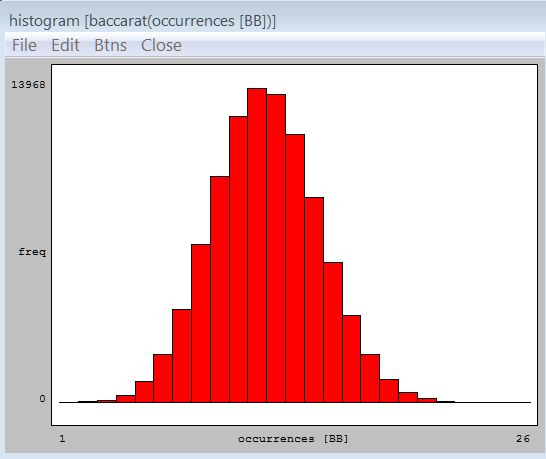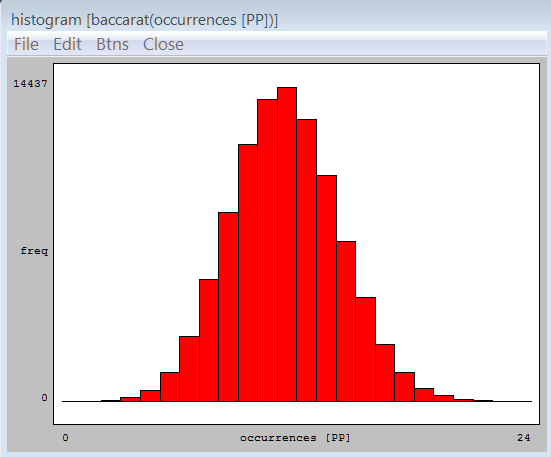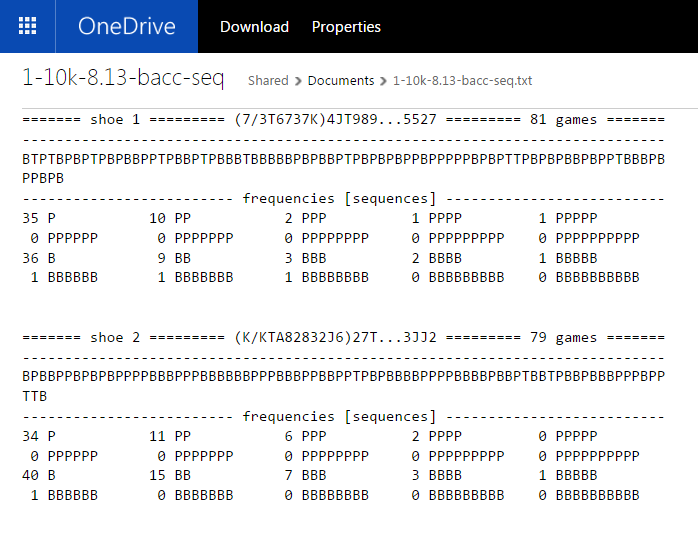mustangsallyJoined: Mar 29, 2011
• Posts: 2463
May 13th, 2015 at 8:22:26 AM permalink
PP and BB sequences per 8 deck shoe
for totals just add the ev and variance like so (totals are in another post)
11.90 + 12.45 = 24.35 (mean)
7.87 + 7.60 = 15.47 (variance)
standard deviation = 3.93
`sequence BB       group         middle    freq  freq/100--------------------------------------------- 1.50 <= x <  2.50     2.00       2     0.00% 2.50 <= x <  3.50     3.00      23     0.02% 3.50 <= x <  4.50     4.00      92     0.09% 4.50 <= x <  5.50     5.00     306     0.31% 5.50 <= x <  6.50     6.00     920     0.92% 6.50 <= x <  7.50     7.00    2133     2.13% 7.50 <= x <  8.50     8.00    4157     4.16% 8.50 <= x <  9.50     9.00    7027     7.03% 9.50 <= x < 10.50    10.00   10058    10.06%10.50 <= x < 11.50    11.00   12705    12.70%11.50 <= x < 12.50    12.00   13968    13.97%12.50 <= x < 13.50    13.00   13694    13.69%13.50 <= x < 14.50    14.00   11923    11.92%14.50 <= x < 15.50    15.00    9114     9.11%15.50 <= x < 16.50    16.00    6217     6.22%16.50 <= x < 17.50    17.00    3846     3.85%17.50 <= x < 18.50    18.00    2113     2.11%18.50 <= x < 19.50    19.00    1004     1.00%19.50 <= x < 20.50    20.00     457     0.46%20.50 <= x < 21.50    21.00     165     0.16%21.50 <= x < 22.50    22.00      57     0.06%22.50 <= x < 23.50    23.00      13     0.01%23.50 <= x < 24.50    24.00       4     0.00%24.50 <= x < 25.50    25.00       1     0.00%25.50 <= x < 26.50    26.00       1     0.00%---------------------------------------------                      grouped dataitems:                  100000   minimum value:               2.00first quartile:             11.00median:                     12.00third quartile:             14.00maximum value:              26.00mean value:                 12.45 <<<<<midrange:                   14.00range:                      24.00interquartile range:         3.00mean abs deviation:          2.26sample variance (n):         7.87sample variance (n-1):       7.87 <<<<<sample std dev (n):          2.81sample std dev (n-1):        2.81---------------------------------------------                 cumulative--------------------------------------------- 1.50 <= x <  2.50     2.00       2     0.00% 2.50 <= x <  3.50     3.00      25     0.02% 3.50 <= x <  4.50     4.00     117     0.12% 4.50 <= x <  5.50     5.00     423     0.42% 5.50 <= x <  6.50     6.00    1343     1.34% 6.50 <= x <  7.50     7.00    3476     3.48% 7.50 <= x <  8.50     8.00    7633     7.63% 8.50 <= x <  9.50     9.00   14660    14.66% 9.50 <= x < 10.50    10.00   24718    24.72%10.50 <= x < 11.50    11.00   37423    37.42%11.50 <= x < 12.50    12.00   51391    51.39%12.50 <= x < 13.50    13.00   65085    65.08%13.50 <= x < 14.50    14.00   77008    77.01%14.50 <= x < 15.50    15.00   86122    86.12%15.50 <= x < 16.50    16.00   92339    92.34%16.50 <= x < 17.50    17.00   96185    96.18%17.50 <= x < 18.50    18.00   98298    98.30%18.50 <= x < 19.50    19.00   99302    99.30%19.50 <= x < 20.50    20.00   99759    99.76%20.50 <= x < 21.50    21.00   99924    99.92%21.50 <= x < 22.50    22.00   99981    99.98%22.50 <= x < 23.50    23.00   99994    99.99%23.50 <= x < 24.50    24.00   99998   100.00%24.50 <= x < 25.50    25.00   99999   100.00%25.50 <= x < 26.50    26.00  100000   100.00%sequence PP       group         middle    freq  freq/100--------------------------------------------- 0.50 <= x <  1.50     1.00       4     0.00% 1.50 <= x <  2.50     2.00       1     0.00% 2.50 <= x <  3.50     3.00      30     0.03% 3.50 <= x <  4.50     4.00     159     0.16% 4.50 <= x <  5.50     5.00     507     0.51% 5.50 <= x <  6.50     6.00    1331     1.33% 6.50 <= x <  7.50     7.00    2976     2.98% 7.50 <= x <  8.50     8.00    5591     5.59% 8.50 <= x <  9.50     9.00    8709     8.71% 9.50 <= x < 10.50    10.00   11829    11.83%10.50 <= x < 11.50    11.00   13900    13.90%11.50 <= x < 12.50    12.00   14437    14.44%12.50 <= x < 13.50    13.00   12970    12.97%13.50 <= x < 14.50    14.00   10407    10.41%14.50 <= x < 15.50    15.00    7373     7.37%15.50 <= x < 16.50    16.00    4782     4.78%16.50 <= x < 17.50    17.00    2622     2.62%17.50 <= x < 18.50    18.00    1351     1.35%18.50 <= x < 19.50    19.00     611     0.61%19.50 <= x < 20.50    20.00     274     0.27%20.50 <= x < 21.50    21.00      91     0.09%21.50 <= x < 22.50    22.00      32     0.03%22.50 <= x < 23.50    23.00      10     0.01%23.50 <= x < 24.50    24.00       3     0.00%---------------------------------------------                      grouped dataitems:                  100000   minimum value:               1.00first quartile:             10.00median:                     12.00third quartile:             14.00maximum value:              24.00mean value:                 11.90 <<<<<midrange:                   12.50range:                      23.00interquartile range:         4.00mean abs deviation:          2.19sample variance (n):         7.60 <<<<<sample variance (n-1):       7.60sample std dev (n):          2.76sample std dev (n-1):        2.76---------------------------------------------                 cumulative--------------------------------------------- 0.50 <= x <  1.50     1.00       4     0.00% 1.50 <= x <  2.50     2.00       5     0.00% 2.50 <= x <  3.50     3.00      35     0.04% 3.50 <= x <  4.50     4.00     194     0.19% 4.50 <= x <  5.50     5.00     701     0.70% 5.50 <= x <  6.50     6.00    2032     2.03% 6.50 <= x <  7.50     7.00    5008     5.01% 7.50 <= x <  8.50     8.00   10599    10.60% 8.50 <= x <  9.50     9.00   19308    19.31% 9.50 <= x < 10.50    10.00   31137    31.14%10.50 <= x < 11.50    11.00   45037    45.04%11.50 <= x < 12.50    12.00   59474    59.47%12.50 <= x < 13.50    13.00   72444    72.44%13.50 <= x < 14.50    14.00   82851    82.85%14.50 <= x < 15.50    15.00   90224    90.22%15.50 <= x < 16.50    16.00   95006    95.01%16.50 <= x < 17.50    17.00   97628    97.63%17.50 <= x < 18.50    18.00   98979    98.98%18.50 <= x < 19.50    19.00   99590    99.59%19.50 <= x < 20.50    20.00   99864    99.86%20.50 <= x < 21.50    21.00   99955    99.96%21.50 <= x < 22.50    22.00   99987    99.99%22.50 <= x < 23.50    23.00   99997   100.00%23.50 <= x < 24.50    24.00  100000   100.00%`

what they look like individually
BBPP==========================
for enjoyment

I added this file to my online folder (link in me blog) one can get the distribution from this file if wants
10,000 shoes to play with

1-10k-8.13-bacc-seq.txt

http://1drv.ms/1A0xunt

it opens fine (in a few seconds and scrolls) in my Google Chrome browser
i do not know about other browser performance